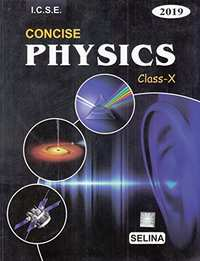Share

# Selina Solutions for Class 10 Physics

## Solutions for Selina ICSE Concise Physics for Class 10

Shaalaa provides Selina Solutions for Class 10 Physics and has all the answers for the questions given in Selina ICSE Concise Physics for Class 10. Shaalaa is surely a site that most of your classmates are using to perform in exams.

You can solve Selina ICSE Concise Physics for Class 10 and use Shaalaa Selina Solutions for Class 10 Physics to check your answers.

#### Selina Solutions for Class 10 Physics## Chapters covered in Selina Solutions for Class 10 Physics

#### Chapter 1: Force

Shaalaa has a total of 142 questions are solved for this Chapter in Class 10 Physics. Chapters covered in Force are Centre of Gravity, Concept of Force, Elementary Introduction of Translational and Rotational Motions, Forces in Equilibrium, Force - Uniform Circular Motion, Moment of a Force, Turning Forces Concept

#### Chapter 2: Work, Energy and Power

Shaalaa has a total of 189 questions are solved for this Chapter in Class 10 Physics. Chapters covered in Work, Energy and Power are Concept of Work, Energy, Power, Concept of Work, Energy, Power (Sum, Numericals ), Different Types of Energy, Work, Energy, Power - Relation with Force

#### Chapter 3: Machines

Shaalaa has a total of 138 questions are solved for this Chapter in Class 10 Physics. Chapters covered in Machines are Concept of Lever, Concept of Machines Numercials, Concept of Pulley System, Functions and Uses of Simple Machines, Machines as Force Multipliers, Principle of Conservation of Energy

#### Chapter 4: Refraction of Light at Plane Surfaces

Shaalaa has a total of 110 questions are solved for this Chapter in Class 10 Physics. Chapters covered in Refraction of Light at Plane Surfaces are Applications of Refraction of Light, Refraction of Light Through a Glass Block and a Triangular Prism, Refraction of Light Through Plane Surface, Total Internal Reflection, Total Internal Reflection - Critical Angle

#### Chapter 5: Refraction through a Lens

Shaalaa has a total of 130 questions are solved for this Chapter in Class 10 Physics. Chapters covered in Refraction through a Lens are Concept of Magnifying Glass, Concept Refraction of Light Through a Lense, Image Formation by Lenses - Convex Lens, Image Formation in Lenses Using Ray Diagrams - Concave Lens, Power of a Lens, Refraction by a Lens, Types of Lenses (Converging and Diverging),

#### Chapter 6: Spectrum

Shaalaa has a total of 89 questions are solved for this Chapter in Class 10 Physics. Chapters covered in Spectrum are Concept of Spectrum, Electromagnetic Spectrum, Some Natural Phenomena Due to Sunlight, Using a Triangular Prism to Produce a Visible Spectrum from White Light

#### Chapter 7: Sound

Shaalaa has a total of 113 questions are solved for this Chapter in Class 10 Physics. Chapters covered in Sound are Concept of Sound, Concept of Sound Numericals, Damped Vibrations, Forced Vibrations and Resonance, Loudness, Pitch and Quality of Sound, Natural Vibrations, Reflection of Sound Waves

#### Chapter 8: Current Electricity

Shaalaa has a total of 92 questions are solved for this Chapter in Class 10 Physics. Chapters covered in Current Electricity are Concept of Current Electricity, Concept of Current Electricity Numericals, Concepts of Emf, Concepts of Pd (V), Current (I), Resistance (R) and Charge (Q)., Electric Potential Difference, Internal Resistance, Ohm’s Law, Resistance of a System of Resistors - Resistors in Parallel, Resistance of a System of Resistors - Resistors in Series

#### Chapter 9: Electrical Power and Household Circuits

Shaalaa has a total of 92 questions are solved for this Chapter in Class 10 Physics. Chapters covered in Electrical Power and Household Circuits are Concept of Electrical Power and Household Circuits Numericals, Electrical Energy and Power, Household Circuits - Colour Coding of Wires, Household Circuits - Earthing;, Household Circuits - Fuses, Household Circuits – Main Circuit, Household Circuits - Safety Precautions, Household Circuits - Switches, Household Circuits - Three-pin Plugs

#### Chapter 10: Electro-Magnetism

Shaalaa has a total of 109 questions are solved for this Chapter in Class 10 Physics. Chapters covered in Electro-Magnetism are Ac Generator, Concept of Electro Magnetism, Concept of Transformer, Electromagnetic Induction, Fleming’s Left Hand Rule, Fleming’S Right Hand Rule, Magnetic Effect of a Current

#### Chapter 11: Calorimetry

Shaalaa has a total of 122 questions are solved for this Chapter in Class 10 Physics. Chapters covered in Calorimetry are Calorimetry - Principle of Method of Mixtures, Calorimetry - Specific Heat Capacity, Concept of Calorimetry, Concept of Calorimetry Numercials:, Heat - Latent Heat, Heat - Loss and Gain of Heat Involving Change of State for Fusion Only

#### Chapter 12: Radioactivity

Shaalaa has a total of 0 questions are solved for this Chapter in Class 10 Physics. Chapters covered in Radioactivity are Concept of Nuclear Fission and Fusion;, Nuclear Energy, Radiation, Radioactivity and Changes in the Nucleus

## Selina Solutions for Class 10 Physics

Class 10 Selina solutions answers all the questions given in the Selina textbooks in a step-by-step process. Our Maths tutors have helped us put together this for our Class 10 Students. The solutions on Shaalaa will help you solve all the Selina Class 10 Physics questions without any problems. Every chapter has been broken down systematically for the students, which gives fast learning and easy retention.

Shaalaa provides free Selina solutions for Class 10 Physics. Shaalaa has carefully crafted Selina solutions for Class 10 Physics that can help you understand the concepts and learn how to answer properly in your board exams. You can also share our link for free Class 10 Physics Selina solutions with your classmates.

If you have any doubts while going through our Class 10 Physics Selina solutions, then you can go through our Video Tutorials for Physics. The tutorials should help you better understand the concepts.

S